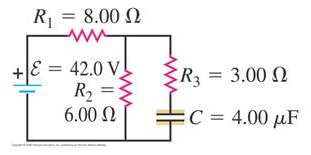# Problem: The capacitor in the figure shown is initially uncharged. The switch is closed at t = 0.Immediately after the switch is closed, what is the current through each resistor?

###### FREE Expert Solution

Equivalent resistance for parallel resistors is

$\overline{)\frac{\mathbf{1}}{{\mathbf{R}}_{\mathbf{e}\mathbf{q}}}{\mathbf{=}}\frac{\mathbf{1}}{{\mathbf{R}}_{\mathbf{1}}}{\mathbf{+}}\frac{\mathbf{1}}{{\mathbf{R}}_{\mathbf{2}}}}$

Equivalent resistance for series resistors:

Ohm's law:

$\overline{){\mathbf{V}}{\mathbf{=}}{\mathbf{i}}{\mathbf{R}}}$

We'll assume that the capacitor has no resistance at t = 0.

R2 and R3 are in parallel:

R23 = (1/R2 + 1/R3)-1 = (1/6.00 + 1/3.00)-1 = 2.00 Ω

R1 is in series with R23:

100% (350 ratings)###### Problem Details

The capacitor in the figure shown is initially uncharged. The switch is closed at t = 0.

Immediately after the switch is closed, what is the current through each resistor?Frequently Asked Questions

What scientific concept do you need to know in order to solve this problem?

Our tutors have indicated that to solve this problem you will need to apply the !! Resistor-Capacitor Circuits concept. If you need more !! Resistor-Capacitor Circuits practice, you can also practice !! Resistor-Capacitor Circuits practice problems.

What professor is this problem relevant for?

Based on our data, we think this problem is relevant for Professor Gandhi's class at UHD.